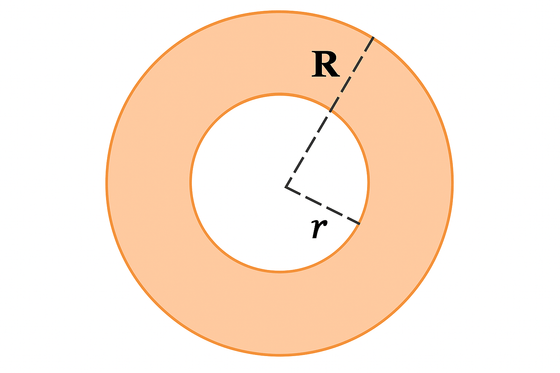# annulus

Annulus
Washer

The region between two concentriccircles which have different radii.

Area of annulus $$= \pi \left( {{R^2} - {r^2}} \right)$$